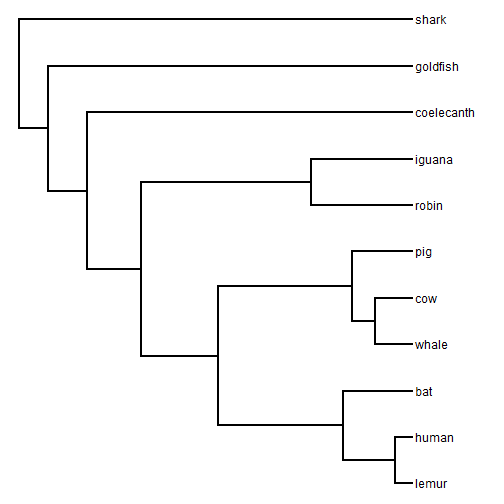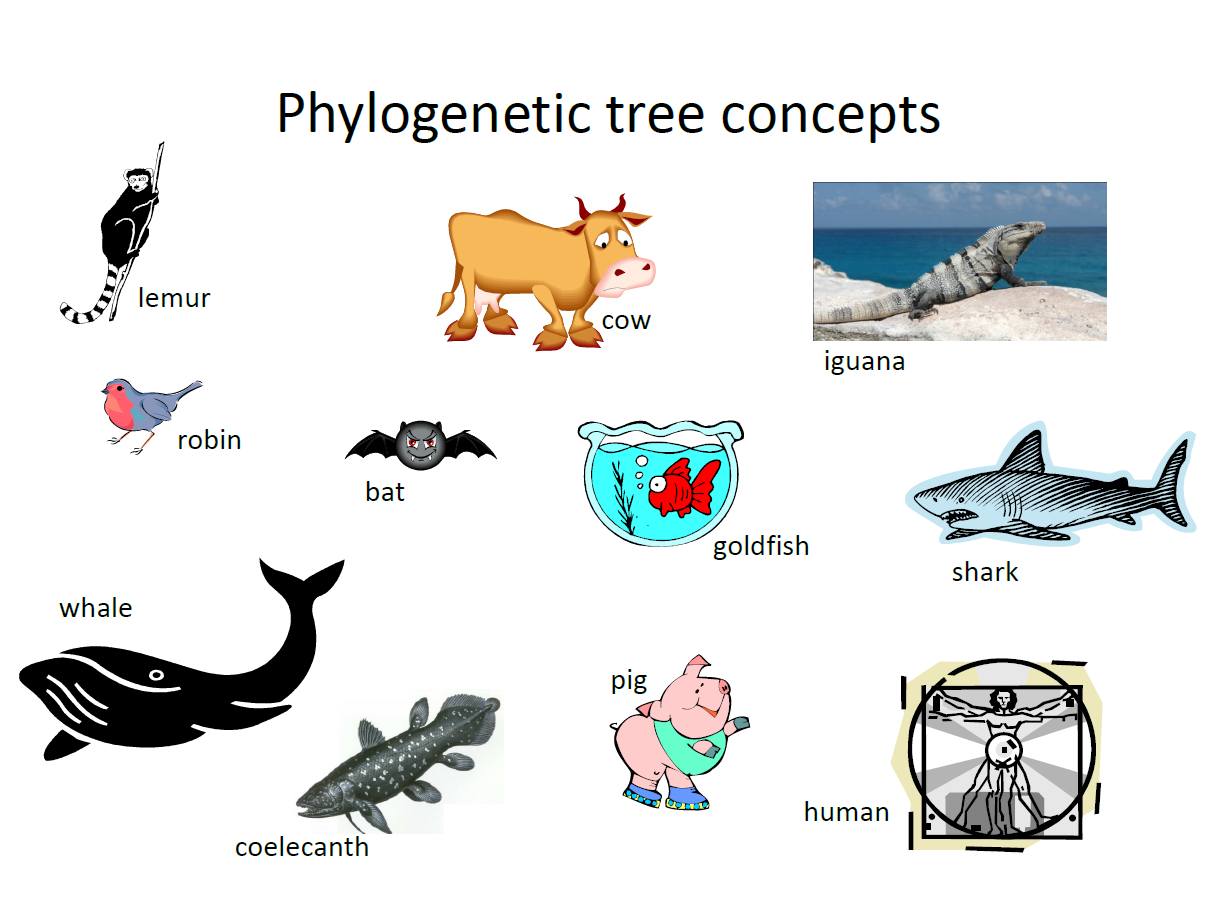## Friday, February 10, 2017

### Additional function for interactive drawing of a cladogram or ultrametric phylogram

I just wrote another function designed to permit users to interactively draw phylogenies in the R environment.

This one is based closely on a demo I posted to my blog a couple of weeks ago showing how to draw a tree using `phytools::bind.tip`. This one uses `bind.tip` in a couple of different ways - either interactively (as in the case for `method="cladogram"` or non-interactively, but via the interactive phytools (mostly internal - although now exported to the namespace) function `get.treepos`.

The following is a quick demo:

``````library(phytools)

tips<-c("lemur","robin","whale","coelecanth","bat","cow",
"goldfish","pig","iguana","human")
tips
``````
``````##   "lemur"      "robin"      "whale"      "coelecanth" "bat"
##   "cow"        "goldfish"   "pig"        "iguana"     "human"
``````
``````outgroup<-"shark"
outgroup
``````
``````##  "shark"
``````
``````draw.ultrametric<-function(ingroup,outgroup=NULL,depth=1.0,
method<-method
if(is.null(outgroup)) out<-"OUTGROUP"
else out<-outgroup
tree<-pbtree(n=2,tip.label=c(ingroup,out),scale=depth)
for(i in 2:length(ingroup))
tree<-bind.tip(tree,ingroup[i],interactive=TRUE)
tree\$edge.length<-NULL
} else if(method=="phylogram"){
dev.hold()
plotTree(tree,mar=c(0.1,0.1,3.1,0.1))
v<-seq(0,depth,by=depth/10)
axis(3,at=v)
abline(v=v,lty="dashed",col=make.transparent("grey",0.7))
dev.flush()
cat(paste("Click where you would like to bind the tip \"",
ingroup,"\"\n",sep=""))
flush.console()
for(i in 2:length(ingroup)){
obj<-get.treepos(message=FALSE)
tree<-bind.tip(tree,ingroup[i],where=obj\$where,
pos=obj\$pos)
dev.hold()
plotTree(tree,mar=c(0.1,0.1,3.1,0.1))
axis(3,at=v)
abline(v=v,lty="dashed",col=make.transparent("grey",0.7))
dev.flush()
if(i<length(ingroup))
cat(paste("Click where you would like to bind the tip \"",
ingroup[i+1],"\"\n",sep=""))
flush.console()
}
}
tree
}
``````
``````vertebrates<-draw.ultrametric(tips,outgroup)
``````
``````plotTree(vertebrates)
``````[The above example is based on one I use in undergrad classes in which I try to get them to come up with a 'phylogeny of vertebrate' from the following slide:from which they are supposed to draw a tree.]

This function will most likely go in the physketch package.

That's all.

#### 1 comment:

1.Note: due to the very large amount of spam, all comments are now automatically submitted for moderation.